Courses

# Dimensions of Physical Quantities- 2

## 10 Questions MCQ Test Physics Class 11 | Dimensions of Physical Quantities- 2

Description
This mock test of Dimensions of Physical Quantities- 2 for JEE helps you for every JEE entrance exam. This contains 10 Multiple Choice Questions for JEE Dimensions of Physical Quantities- 2 (mcq) to study with solutions a complete question bank. The solved questions answers in this Dimensions of Physical Quantities- 2 quiz give you a good mix of easy questions and tough questions. JEE students definitely take this Dimensions of Physical Quantities- 2 exercise for a better result in the exam. You can find other Dimensions of Physical Quantities- 2 extra questions, long questions & short questions for JEE on EduRev as well by searching above.
QUESTION: 1

### Identify the pair whose dimensions are equal. [AIEEE 2002]

Solution:

The dimensions of torque and work are [ML2T-2]

QUESTION: 2

### The physical quantities not having same dimensions are [AIEEE 2003]

Solution:

Planck's constant (in term of unit)
(h) = j-s
= [ML2T-2][T]
= [ML2T-1]
Momentum (p) = kg-ms-1
=[M][L][T-1] = [MLT-1]

QUESTION: 3

### Which of the following is a dimensional constant?

Solution:

The physical quantities which possess dimensions and have constant values are called dimensional constants. Hence Gravitational constant is a dimensional constant.

QUESTION: 4

Dimensions of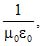where symbols have their usual meaning, are

[AIEEE 2003]

Solution:

As we know that formula of velocity is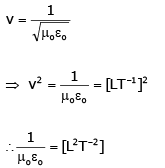QUESTION: 5

Which one of the following represents the correct dimensions of the coefficient of viscosity?

[AIEEE 2004]

Solution:

By Newton's formula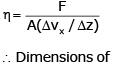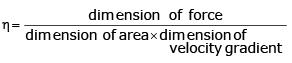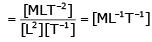QUESTION: 6

The average life of an Indian is 56 years. Find the number of times the human heart beats in the life of an Indian, if the heat beats once in 0.8 s.

Solution:

Average life of an Indian = 56 years = 56×3 65.25 × 24 × 60 × 60 s
Period of heart beat = 0.8 s
Total number of heartbeats in 56 years = (56 × 365.25 × 24 × 60 × 60)/0.8 = 2.2 × 109 times.

QUESTION: 7

Out of the following pairs, which one does not have identical dimensions ?

[AIEEE 2005]

Solution:

I = mr2
∴[I] = [ML2]
and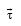= moment of force =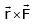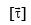= [L][MT-2]=[ML2T-2]

QUESTION: 8

Which of the following units denotes the dimensions [ML2/Q2], where Q denotes the electric charges ?

[AIEEE 2006]

Solution:

Magnetic energy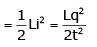[L→ inductance, i → current]
Energy has the dimension = [ML2T-2]
Equate the dimension, we have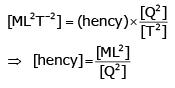QUESTION: 9

A body of mass m = 3.513 kg is moving along the x-axis with a speed of 5.00 ms-1. The magnitude of its momentum is recorded as

[AIEEE 2008]

Solution:

m = 3.513 kg and v = 5.00 ms-1
So momentum, p = mv = 17.565 As the number of significant digits in m is 4 and in v is 3, so p must have 3 (minimum) significant digits.
Hence, p = 17.6 kg ms-1

QUESTION: 10

The dimensions of magnetic field in M, L, T and C (coulomb) is given as

[AIEEE 2008]

Solution:

We know that F = q v B where q is charge, v is velocity and B is magnetic field.
Hence we get B = F / q v
Therefore [B] = [F] / [q] [v]
= MLT-2 / C.LT-1
= MCT-1